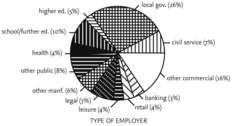# pie chart

(redirected from pie charts)
Also found in: Dictionary, Thesaurus, Medical, Financial.

## pie chart

[′pī ‚chärt]
(mathematics)
A circle divided by several radii into sectors whose relative areas represent the relative magnitudes of quantities or the relative frequencies of items in a frequency distribution. Also known as circle graph; sectorgram.Fig. 23 Pie graph or pie chart. In the above example, sectors of the graph indicate the type of employment of Polytechnic and College social science graduates, three years after graduation.

or

## pie chart

a circular graph divided into sectors proportional to the magnitudes of the quantities represented.

## pie chart

A graphical representation of information in which each unit of data is represented as a pie-shaped piece of a circle. See business graphics.
References in periodicals archive ?
Of course, a pie chart is not necessary in most scientific papers.
One-year follow-up status of patients with acute lymphoblastic leukemia treated with a combination of vincristine and cytarabine Men Women Complete remission (n = 21) (n = 44) Partial remission (n = 52) (n = 41) No change (n = 40) (n = 22) Death from complications (n = 6) (n = 4) Note: Table made from pie chart.
These observations might be of mere academic interest except that studies have shown that not all observers interpret pie charts in the same way, One study, for example, found that 51 percent of pie chart viewers focused on the arcs of their sectors, 23 percent evaluated them according to their central angles, and only 25 percent interpreted them according to their areas.
When all these design features are combined--when pie charts include too many categories, are rotated, exploded, and presented using colors--the result can be graphics that are confusing, like Panel A of Exhibit 2.
Domain level identity pie chart. The fourth instrument used to measure identity was the Parenting Domain Pie Chart, developed for this study.
What is the relationship between pie chart and scale measures of identity?
Graphs take many forms, but accounting information is usually presented in one of three types: the bar graph, the line plot and the pie chart. Almost any accounting data can be shown graphically--inventories, receivables, sales, gross profits, or net income.
This graph can show parts of a whole (like the pie chart) for several entities at the same time.
Write pie chart on the chalkboard and have students discuss what a pie chart looks like.
After all circles are drawn and timelines are left on the paper, ask the students to suggest ways to use their timelines to make a pie chart. They will certainly suggest drawing line segments to separate the various activities on their timelines.
where B's are coefficients from logit equation |4~ estimated from responses obtained using the risk ladder and the A's are coefficients from logit equation |4~ estimated from responses obtained using the pie charts.
The best examples of using pie charts to indicate the relative significance of items are for revenues and expenses.

Site: Follow: Share:
Open / Close